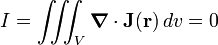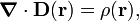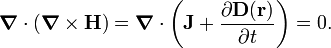# Displacement current

In physics, more particular in the theory of electromagnetism, the displacement current is the time derivative of the electric displacement D (a vector field closely related to the electric field E). The concept was introduced by James Clerk Maxwell in 1861.

A few years after he had introduced the displacement current, Maxwell realized from the analogy of electricity with an incompressible fluid that the displacement current is necessary to describe conservation of charge. At present, charge conservation, as expressed by the continuity equation, is applied routinely in textbooks to extend Ampère's law to one of the four Maxwell equations. The extension will be discussed below.

Ampère's law states that an electric current through a wire causes a magnetic field encircling the wire. In a very similar way, a displacement current (a changing electric field) causes a magnetic field encircling it. It is the displacement current in the extension of Ampère's law that gives rise to electromagnetic waves. This fact was already noticed by Maxwell himself (1865).

## ExplanationCharging of a parallel-plate capacitor (green plates) with current I. V indicates a volume that contains one plate and is cut by the wire.

In the figure on the right a capacitor (with green plates) is charged by an electric current I. The conduction current I is related to the current density J(r) by a surface integral. Since J is everywhere zero, except over the surface of the wire (blue in the figure), one may integrate over the whole closed surface of the volume V (see the figure) to get I,$I = \iint_{\mathrm{closed}\atop\mathrm{surface}} \mathbf{J}(\mathbf{r})\cdot d\mathbf{S} = \iiint_V \boldsymbol{\nabla} \cdot \mathbf{J}(\mathbf{r})\, dv,$

where the last equality follows by application of the divergence theorem of Gauss. The surface integral contains the inner product between the current density J (a vector) and a vector dS orthogonal to the infinitesimal surface element dS. The orthogonal vector has length dS. The conduction current I gives the rate of change of electric charge in the volume V. Clearly, I is non-zero during the time that the capacitor is charging.

Ampère's law in differential form states that (in SI units)$\boldsymbol{\nabla} \times \mathbf{H}(\mathbf{r}) = \mathbf{J}(\mathbf{r}),$

where H(r) is the magnetic field. Since the divergence of the curl is always zero,$\boldsymbol{\nabla}\cdot(\boldsymbol{\nabla} \times \mathbf{H}) = \boldsymbol{\nabla}\cdot \mathbf{J}(\mathbf{r}) = 0,$

it follows that$I = \iiint_V \boldsymbol{\nabla} \cdot \mathbf{J}(\mathbf{r})\, dv = 0$

at all times, in contradiction to what was just stated. Thus, Ampère's law is in error, or at least is incomplete.

Maxwell was the first to notice this. The problem can be fixed by application of the continuity equation. This equation states that the rate of change of a charge density is proportional to the the divergence of the current density,$\boldsymbol{\nabla}\cdot \mathbf{J} + \frac{\partial \rho}{\partial t} = 0.$

The electrostatic law of Gauss states$\boldsymbol{\nabla}\cdot \mathbf{D}(\mathbf{r}) = \rho(\mathbf{r}),$

so that$\frac{\partial \rho}{\partial t} = \boldsymbol{\nabla}\cdot \frac{\partial\mathbf{D}(\mathbf{r})}{\partial t}, \qquad\hbox{and}\qquad \boldsymbol{\nabla}\cdot\left( \mathbf{J} + \frac{\partial\mathbf{D}(\mathbf{r})}{\partial t} \right) = 0.$

About ten years after his introduction of the displacement current, Maxwell realized that the conduction current leaving the volume V was compensated by the displacement current entering V. The displacement current may be added to the conduction current density, modifying Ampère's law to$\boldsymbol{\nabla} \times \mathbf{H}(\mathbf{r}) = \mathbf{J}(\mathbf{r}) + \frac{\partial\mathbf{D}(\mathbf{r})}{\partial t}, \qquad\qquad\qquad\qquad\qquad\qquad(1)$

with$\boldsymbol{\nabla}\cdot(\boldsymbol{\nabla} \times \mathbf{H}) = \boldsymbol{\nabla}\cdot\left( \mathbf{J}+ \frac{\partial\mathbf{D}(\mathbf{r})}{\partial t} \right) = 0.$

Equation (1) is one of Maxwell's equations formulated by him in 1865 and still accepted as universally valid. The equation is in SI units; in Gaussian units it reads$\boldsymbol{\nabla} \times \mathbf{H}(\mathbf{r}) = \frac{4\pi}{c}\mathbf{J}(\mathbf{r}) + \frac{1}{c} \frac{\partial\mathbf{D}(\mathbf{r})}{\partial t},$

with c the speed of light.

### Note

The term "displacement current" was introduced by Maxwell and is still in general use. However, the term "displacement current density" would be more appropriate. One can define the total displacement current passing through a closed surface S,$I_\mathrm{displ} \equiv \iint_S \frac{\partial\mathbf{D}}{\partial t}\cdot d\mathbf{S}$

in the same way as the conduction current through the same closed surface,$I \equiv I_\mathrm{cond} = \iint_S \mathbf{J}\cdot d\mathbf{S}.$

From$\boldsymbol{\nabla}\cdot\left( \mathbf{J}+ \frac{\partial\mathbf{D}(\mathbf{r})}{\partial t} \right) = 0$

and the divergence theorem follows$I_\mathrm{cond} = - I_\mathrm{displ} \,.$

Thus, the conduction current leaving the volume enveloped by the closed surface S is equal to the total displacement current entering this volume. The displacement current shares this property with the conduction current, although there is no motion of charge involved in the former.